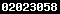# Manually Work Out Single Bets.HOME## How to Work Out Your Single Bets Manually - Quick and Simple Way To Calculate Your Fractional Win Bets.

This article will explain how to work out your win single fractional bets very quickly,manually - no need to have access to calculator ( by calculator is another article Work Out Single Bets By Calculator).

This article also only concerns fractional bets - decimal bets on a separate article.

Work Out Your WIN SP or fraction bets manually.

It is best to just use some real fractional odds and then explain the procedures - it is pretty simple!.

To get to the equation to work out your bet you need to look at the odds 6/4.

Then equalise the right hand number and the left hand number - by that i mean the 4 must equal 6.

How ? - For 4 to equal 6 you need to add the original 4 with 2 ( to equal 6).

In other words,evens (the original 4) plus half (as 2 is half of the original 4).

So,6/4 is simply Evens (that 4) + Half (2 which is half of the 4).

To work out £1 on 6/4 = 1 (this 1 represents your original stake) + 1 +.5 = 2.5 (i.e.£2.50).

Maybe you have £5 at 6/4 - exactly the same procedure,but this time you start equation with 5 (or any other stake unit you have).

£5 at 6/4 = 5 + 5 + 2.5 = £12.50......More difficult price this time 11/8.

Again you need to equalise the left hand and right hand numbers.

So,8 needs to be 11 - which is 8 + 2 + 1 which makes a total of 11.

Which gives you a manual fraction to use of....................Evens (the original 8) + ¼ (which is value of 2 which is a ¼ of 8 ) + half (which is half of that ¼ of 8).

As you can see,you always need to use the previous figure when working out value at each stage.

So,11/8 = Evens (original stake of £1) + Evens (making 8) + ¼ (making 10) + half (making 11,which is what we need to reach 11/11).

So,fractions for 11/8 = evens + ¼ + ½.

So £1 at 11/8 = 1 + 1 + .25 + .125 = £2.375 rounded up to £2.38.

£4 at 11/8 = 4 + 4 + 1 + .50 = £9.50.

This time 15/8 odds.

Sometimes you need to look at the fraction before proceeding.

You can work out 15/8 as evens + ½ + ½ + ½ (1 + 1 +.50 + .25 + .12 = £2.87) using procedures adopted for 6/4 and 11/8 above.

But you can take short cut with this price - look at 15/8 - it is 2/1 minus 1 eighth !.

So,1 multiplied by 3 minus 1 eighth (and remember you use the original number,not the previous number this time when deducting).

So,£1 at 15/8 = 1 times 3 minus .125 = £2.87.

You would use similar short cuts for prices like 11/4,which is 3/1 (12/4) minus ¼ (1 times 4 = 4 minus ¼ of stake which is .25) = 1 times 4 minus .25 = £3.75.

Same for odds on shots - use 8/13.

This time you need to recognise the relationship of first number with second number.

So,go to nearest fraction that makes sense - in this case 8/12 (which is 4/6) and then adjust to 8/13

So,8/13 = 4/6 minus 1/13th.

Looks horrendous at first,but not too hard....

You know 2/3rds of 1 is .67,so you just need to deduct 1/13th of that.

£1 at 8/13 = 1 + .67 minus 5 (which 1/13th of .67) = £1.62.

Just multiply the £1.62 by your bet stake.

One more odds on shot - 8/15.

Again,look at these odds 8/15 is almost 8/16 - which is 1/2 (use 1/2 then add 1/15th).

So,8/15 is actually 1 + .50 + .03(1/15th of .50) = £1.53.

It can seem daunting at first,but try some prices,with differing stakes,and do a quick check using Work Out Single Bets By Calculator advice,and the more you do the easier it gets.

By Pendil at SAFE BOOKMAKERS SITES.©This article can be reproduced by anyone,so long as article is reproduced full and intact with all links unchanged.End of article.

.

 Gambling is fun and a great hobby,but sometimes it can get out of hand - if you need help please contact begambleaware now.